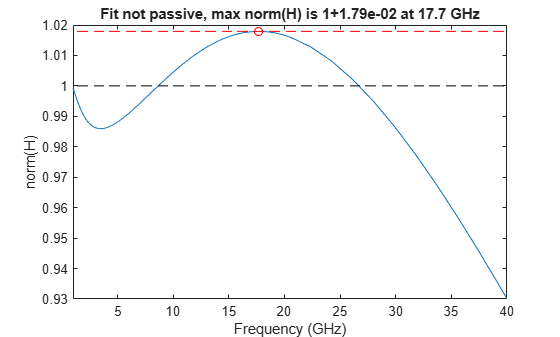# ispassive

Return true if `rationalfit` output is passive at all frequencies

## Syntax

``result = ispassive(fit)``
``[result,maxfreq,maxvalue] = ispassive(fit)``

## Description

example

````result = ispassive(fit)` determines if the rational fit input, `fit` is passive at all frequencies (0,Inf).```
````[result,maxfreq,maxvalue] = ispassive(fit)` determines if the rational fit input, `fit` is passive at all frequencies (0,Inf).```

## Examples

collapse all

Read the file, `passive.s2p` and fit the 2-by-2 S-parameters.

```S = sparameters('passive.s2p'); fit = rationalfit(S);```

Test the passivity of the 2-by-2 fit.

`ispassive(fit)`
```ans = logical 0 ```

Plot the passivity of the 2-by-2 fit.

```figure passivity(fit,[1e9 40e9])```## Input Arguments

collapse all

Rational fit object, specified as an N-by-N array of `rfmodel.rational` objects as returned by the `rationalfit` function or a `rational` object.

## Output Arguments

collapse all

Result of passivity test of the `rfmodel.rational` object, returned as `true` or `false`. The result tells you if the `fit` can result in unstable simulations.

Frequency at which norm(H) is equal to maximum value, returned as a scalar in hertz.

Maximum norm(H) over frequencies (0,Inf), returned as a scalar.

## Version History

Introduced in R2010a